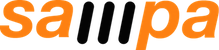## DECAF: Probability Types

Approximate computing needs programming systems that can bound the chance that a result will be wrong. DECAF (DECAF, an Energy-aware Compiler to make Approximation Flexible) is a language that uses probability types to control incorrectness.

### Paper

Probability Type Inference for Flexible Approximate Programming. Brett Boston, Adrian Sampson, Dan Grossman, and Luis Ceze. To appear in OOPSLA 2015.

(We’ll post a link to the final version of the paper when it’s ready.)

## Source Code

The source code for the compiler, simulator, and benchmarks is available for download. The compiler and simulator are modified versions of the EnerJ infrastructure. You will need three bundles of source code in total:

### Building

To build the system, follow the instructions for building EnerJ with the following differences:

• Use version 1.8.0 of the Checker Framework. (The original EnerJ compiler uses an older version.)
• To tell DECAF where to find the Checker Framework, set your `CHECKERFRAMEWORK` environment variable to point to the directory containing the “checker” directory. (Note that this differs from the `JSR308` variable used by the original EnerJ version.)
• You will need Z3. We used version 4.3.1 in our experiments.

## Result Data

You can download a tarball of JSON files if you’re interested in using the statistics described in the paper’s evaluation.

### Main Results

The main results in the DECAF paper consist of the frequency of different “probability levels” among the operations in the benchmarks. In the paper, the data is shown in Figure 3. The full details for these counts are compiled in the file `level_breakdown.json`.

The data file is a JSON document containing an object whose keys are the benchmarks in the evaluation. Each benchmark is associated with an object with these keys:

• The numbers 2 through 9: These reflect the 8 machine architecture configurations we studied, each with a different number (from 2 to 9) of discrete probability levels for each operation. The JSON object for each of these keys gives the proportion of dynamic operations that ran at each probability level.
• “continuous”: A similar object reflecting an idealized machine with no discrete probability levels, i.e., the DECAF compiler is free to choose arbitrary distinct probabilities for every operation in the program.
• “asterisks”: By default, the discrete-level configurations are generated by a version of DECAF configured to solve for the hardware profile a priori. When this is intractable, we fall back to rounding after the fact. The “asterisks” list indicates the configurations where this fallback occurred. It corresponds to the asterisks along the x-axis in Figure 3.

### Raw Benchmark Data

The tarball also contains the raw data for each benchmark. There are two files per benchmark: `*.continuous.json` and `*.discrete.json`. The former reflects unconstrained runs (i.e., no level-solving) and the latter shows runs with solved probability levels (when this was tractable). The former also contains data for when the continuous levels are rounded post facto.

Each element in the data files corresponds to a configuration of the benchmark. Each configuration contains the number of approximate operations per probability level (“approx”), the number of precise operations (“precise”), the number of dynamically-tracked operations (“dyn”), and the total time in seconds it took to compile the benchmark (“build_time”).